# DERIVATIVES OF INVERSE TRIG FUNCTIONS To get derivatives

• Slides: 13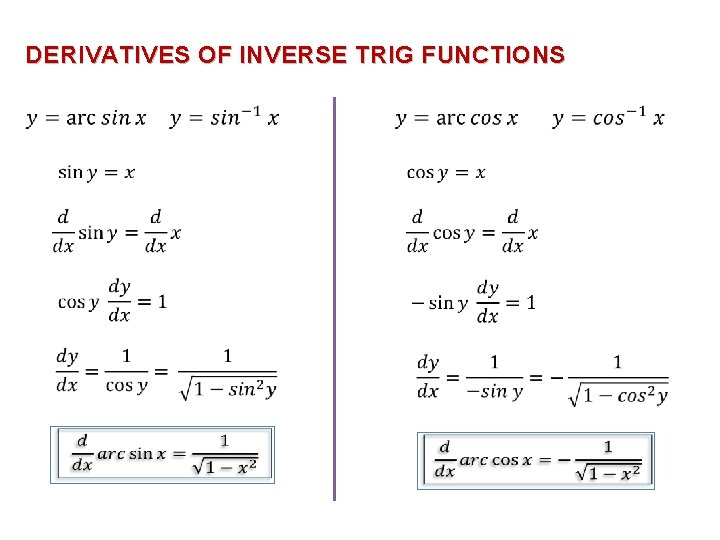DERIVATIVES OF INVERSE TRIG FUNCTIONS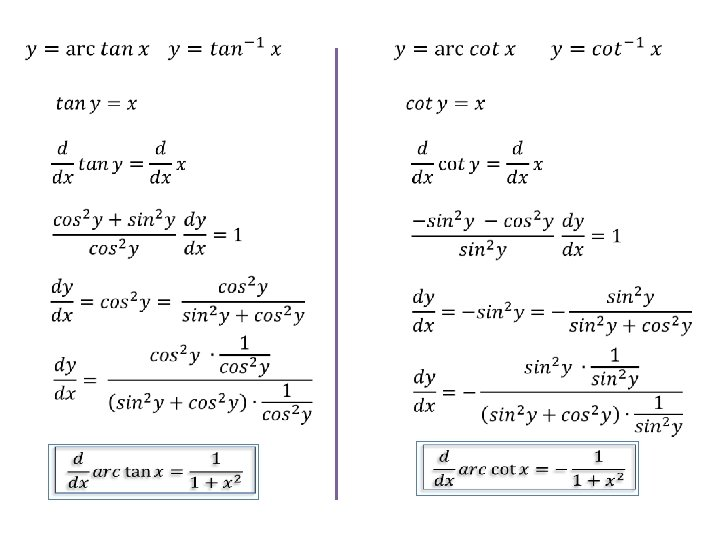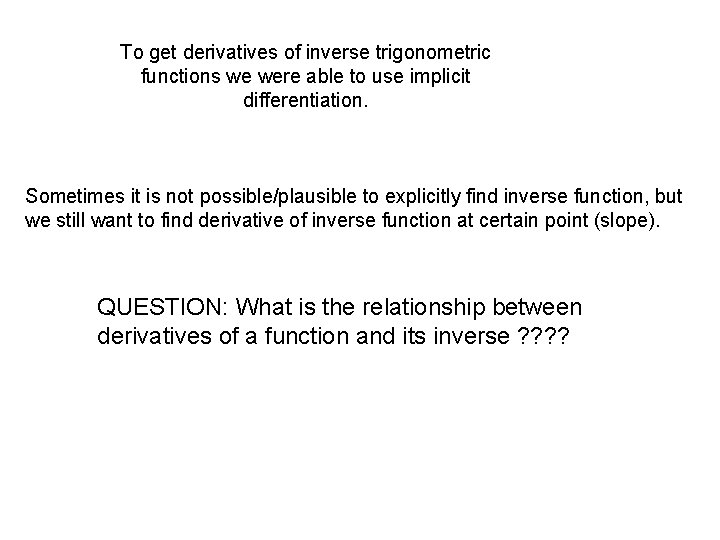To get derivatives of inverse trigonometric functions we were able to use implicit differentiation. Sometimes it is not possible/plausible to explicitly find inverse function, but we still want to find derivative of inverse function at certain point (slope). QUESTION: What is the relationship between derivatives of a function and its inverse ? ?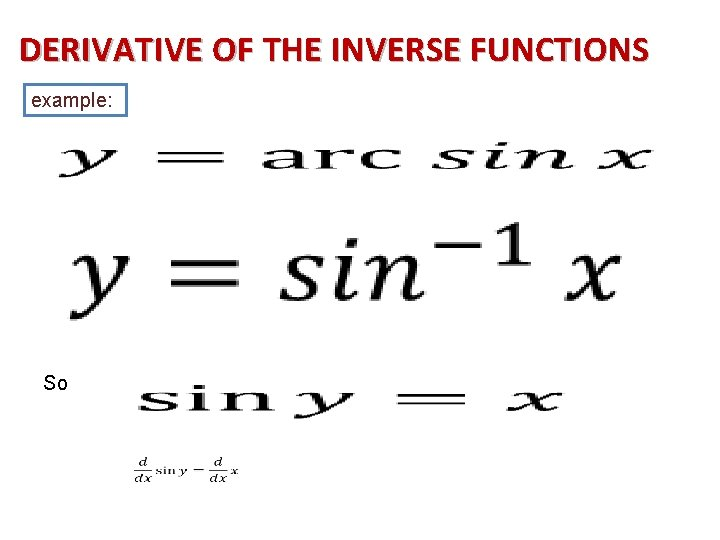DERIVATIVE OF THE INVERSE FUNCTIONS example: So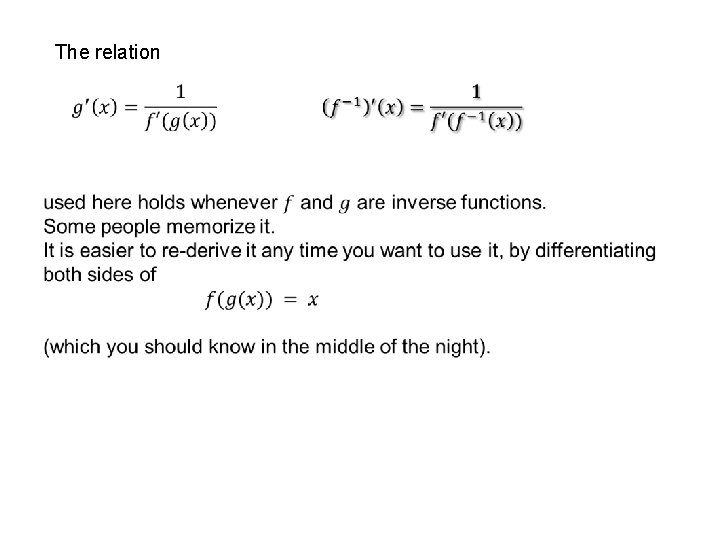The relationexample: A typical problem using this formula might look like this: Given: Find: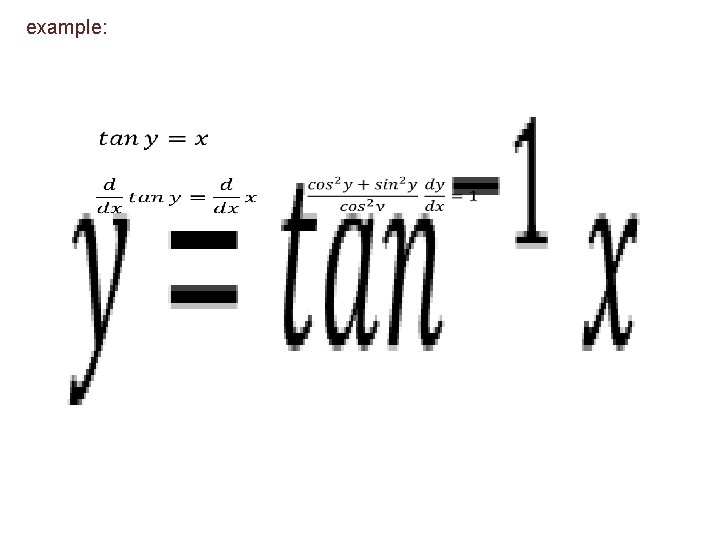example: •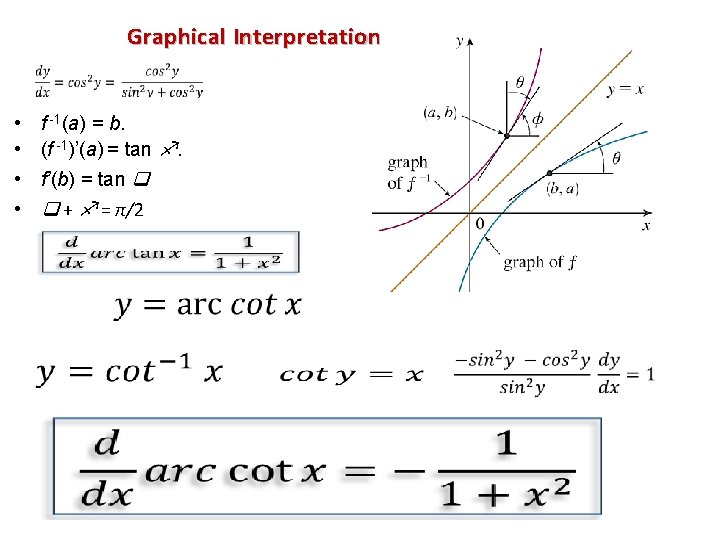Graphical Interpretation • • f -1(a) = b. (f -1)’(a) = tan . f’(b) = tan + = π/2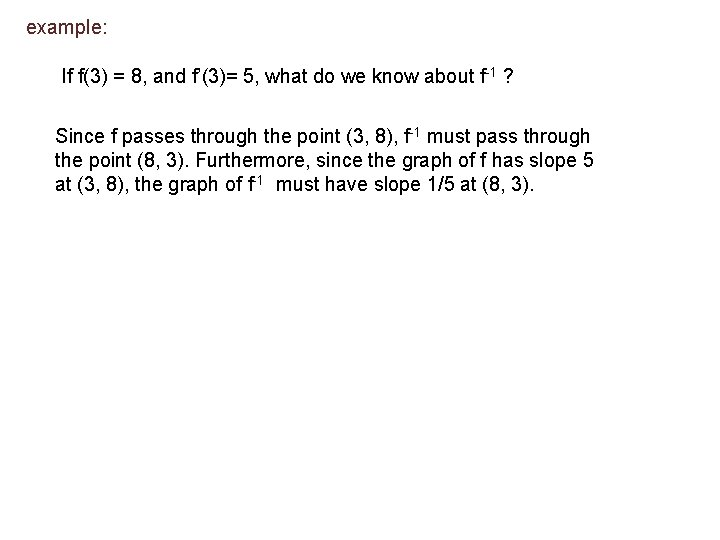example: If f(3) = 8, and f’(3)= 5, what do we know about f-1 ? Since f passes through the point (3, 8), f-1 must pass through the point (8, 3). Furthermore, since the graph of f has slope 5 at (3, 8), the graph of f-1 must have slope 1/5 at (8, 3).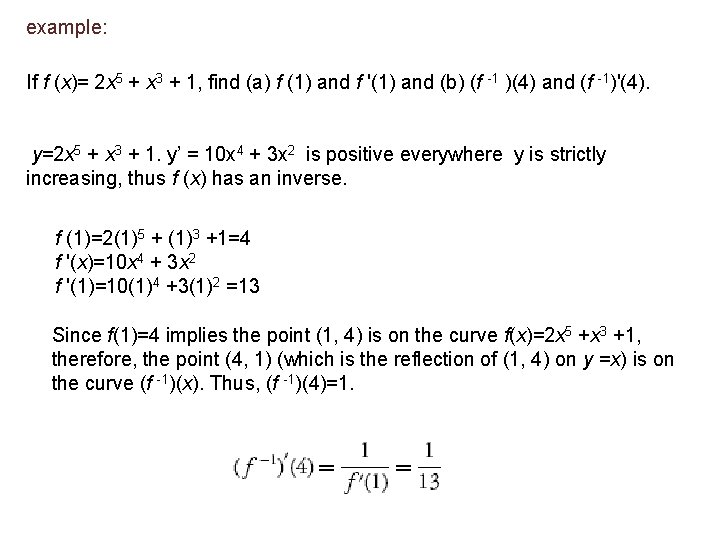example: If f (x)= 2 x 5 + x 3 + 1, find (a) f (1) and f '(1) and (b) (f -1 )(4) and (f -1)'(4). y=2 x 5 + x 3 + 1. y’ = 10 x 4 + 3 x 2 is positive everywhere y is strictly increasing, thus f (x) has an inverse. f (1)=2(1)5 + (1)3 +1=4 f '(x)=10 x 4 + 3 x 2 f '(1)=10(1)4 +3(1)2 =13 Since f(1)=4 implies the point (1, 4) is on the curve f(x)=2 x 5 +x 3 +1, therefore, the point (4, 1) (which is the reflection of (1, 4) on y =x) is on the curve (f -1)(x). Thus, (f -1)(4)=1.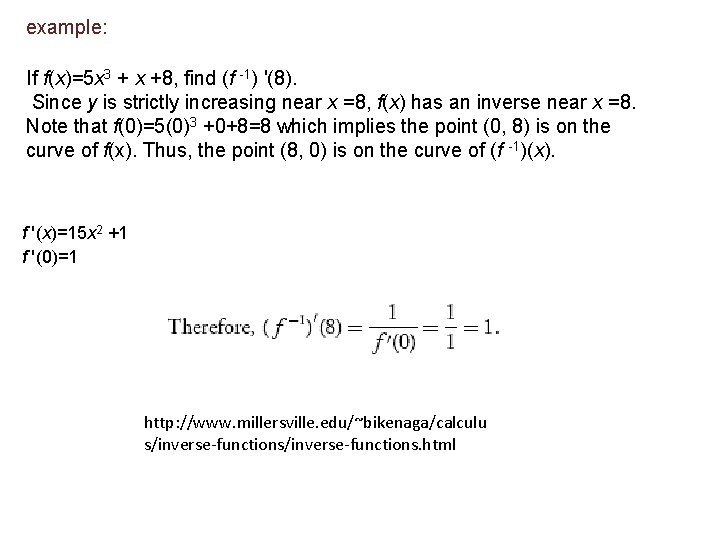example: If f(x)=5 x 3 + x +8, find (f -1) '(8). Since y is strictly increasing near x =8, f(x) has an inverse near x =8. Note that f(0)=5(0)3 +0+8=8 which implies the point (0, 8) is on the curve of f(x). Thus, the point (8, 0) is on the curve of (f -1)(x). f '(x)=15 x 2 +1 f '(0)=1 http: //www. millersville. edu/~bikenaga/calculu s/inverse-functions. html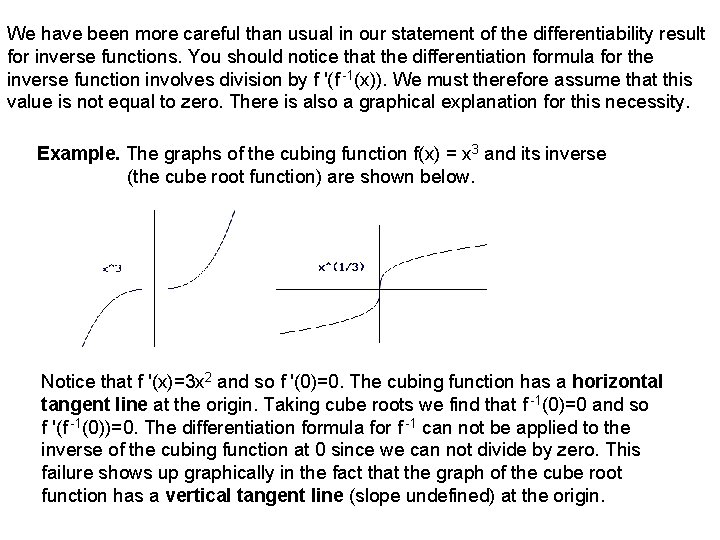We have been more careful than usual in our statement of the differentiability result for inverse functions. You should notice that the differentiation formula for the inverse function involves division by f '(f -1(x)). We must therefore assume that this value is not equal to zero. There is also a graphical explanation for this necessity. Example. The graphs of the cubing function f(x) = x 3 and its inverse (the cube root function) are shown below. Notice that f '(x)=3 x 2 and so f '(0)=0. The cubing function has a horizontal tangent line at the origin. Taking cube roots we find that f -1(0)=0 and so f '(f -1(0))=0. The differentiation formula for f -1 can not be applied to the inverse of the cubing function at 0 since we can not divide by zero. This failure shows up graphically in the fact that the graph of the cube root function has a vertical tangent line (slope undefined) at the origin.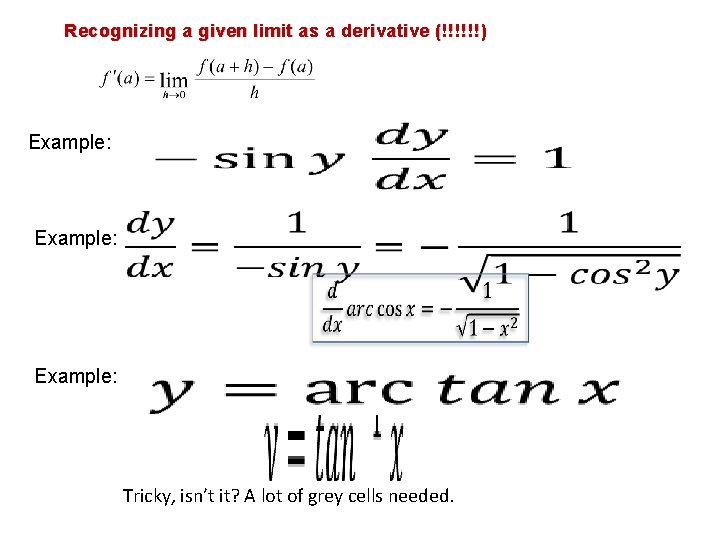Recognizing a given limit as a derivative (!!!!!!) Example: Tricky, isn’t it? A lot of grey cells needed.